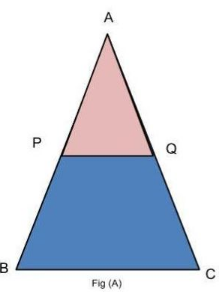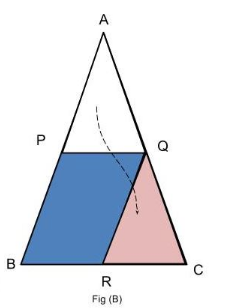# Theory - To verify the mid-point theorem for a triangle, Math, Class 9 Class 9 Notes | EduRev

## Class 9 : Theory - To verify the mid-point theorem for a triangle, Math, Class 9 Class 9 Notes | EduRev

The document Theory - To verify the mid-point theorem for a triangle, Math, Class 9 Class 9 Notes | EduRev is a part of the Class 9 Course Mathematics (Maths) Class 9.
All you need of Class 9 at this link: Class 9

Objective:

To verify the mid-point theorem for a triangle.

Theorem :

The line segment connecting the midpoints of  two sides of a triangle is parallel to the third side and is congruent to one half of the third side.

Basic concepts and facts

1.Parallel Lines:
Two lines are parallel if they do not meet at any point.
2.Congruent Triangles:
Two triangles are congruent if their corresponding angles and corresponding sides are equal.
3.Similar triangles:
Two triangles are similar if their corresponding angles equal and their corresponding sides are in proportion.

Proof of theorem:Given in the figure A :

AP=PB, AQ=QC.

To prove:

PQ || BC and PQ=1/2 BC

Plan:

To prove ▲ APQ ≅ ▲ QRC

Proof steps:

1. AQ=QC [midpoint]

2. ∠ APQ = ∠QRC [Corresponding angles for parallel lines cut by an transversal].

3. ∠PBR=∠QRC=∠APQ [Corresponding angles for parallel lines cut by an transversal].

4. ∠RQC=∠PAQ [When 2 pairs of corresponding angles are congruent in a triangle, the third pair is also congruent.]

5. Therefore , ▲APQ ≅ ▲QRC

6. AP=QR=PB and PQ=BR=RC.

Since midpoints are unique, and the lines connecting points are unique, the proposition is proven.

Offer running on EduRev: Apply code STAYHOME200 to get INR 200 off on our premium plan EduRev Infinity!

## Mathematics (Maths) Class 9

190 videos|233 docs|82 tests

,

,

,

,

,

,

,

,

,

,

,

,

,

,

,

,

,

,

,

,

,

,

,

,

,

,

,

;